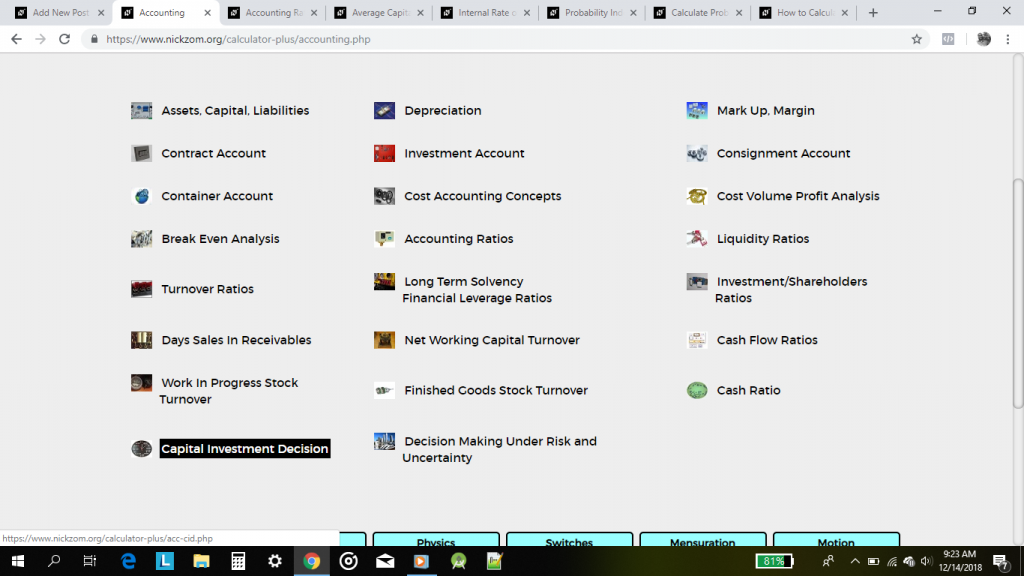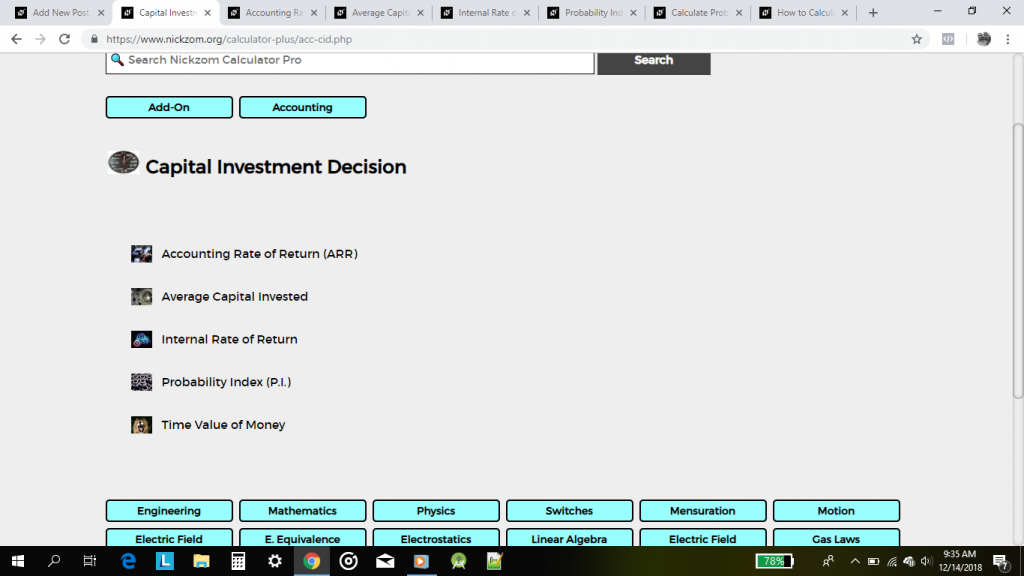## How to Calculate Accounting Rate of Return, Average Capital Invested, Internal Rate of Return, Probability Index and Time Value of Money for Capital Investment Decision

For every Accountant out there, Nickzom Calculator (The Calculator Encyclopedia) can help you get answers to calculations in accounting that can influence your capital investment decisions.

The primary capital investment decisions are:

• Accounting Rate of Return
• Average Capital Invested
• Internal Rate of Return
• Probability Index
• Time Value of Money

As an accountant presenting a report to a firm or for research purposes in a means to influence the decision for capital investment. A proper knowledge and accurate result of the calculations would factor in majorly.

You might be wondering how can I get this Nickzom Calculator and access this functionality?

You can access Nickzom Calculator via any of these means:
Web – https://www.nickzom.org/calculator-plus
Apple (Paid) – https://itunes.apple.com/us/app/nickzom-calculator/id1331162702?mt=8

Once you have got the software, proceed to the Calculator Map, then click on Accounting under the Add-On section.Then, click on Capital Investment Decision in the Accounting category.## The Calculator For Accountants

Nickzom Calculator+ is a 100% all in one package that solves over 32,000 calculations and shows the steps of the calculations. One of the sections it handles is Accounting.
Nickzom Calculator+ solves over 1,500+ calculations in Accounting and shows the steps, formulas, parameters and answer accurately and comprehensively.

Nickzom Calculator+ handles the following major chapters of Accounting:

– Assets, Capital and Liabilities
– Depreciation
– Mark Up, Margin
– Contract Account
– Investment Account
– Consignment Account
– Container Account
– Cost Accounting Concepts
– Cost Profit Volume Analysis
– Break Even Analysis
– Accounting Ratios
– Liquidity Ratios
– Turnover Ratios
– Long Term Solvency Financial Leverage Ratios
– Investment / Shareholders Ratios
– Days Sales in Receivables
– Net Working Capital Turnover
– Finished Goods Stock Turnover
– Cash Ratio
– Capital Investment Decision
– Decision Making Under Risk and Uncertainty

Within these major topics there are sub topics right up to selections of the various parameters you desire to find.

To obtain this calculator via web

Visit https://www.nickzom.org/calculator
Scroll to the Bottom
Click on Access Our Professional Version
Register with Us
Make a Payment of NGN 1,500
Select Accounting in the Drop Down
Click on “Open Section”

To obtain this calculator via Google Play Store (For Android)